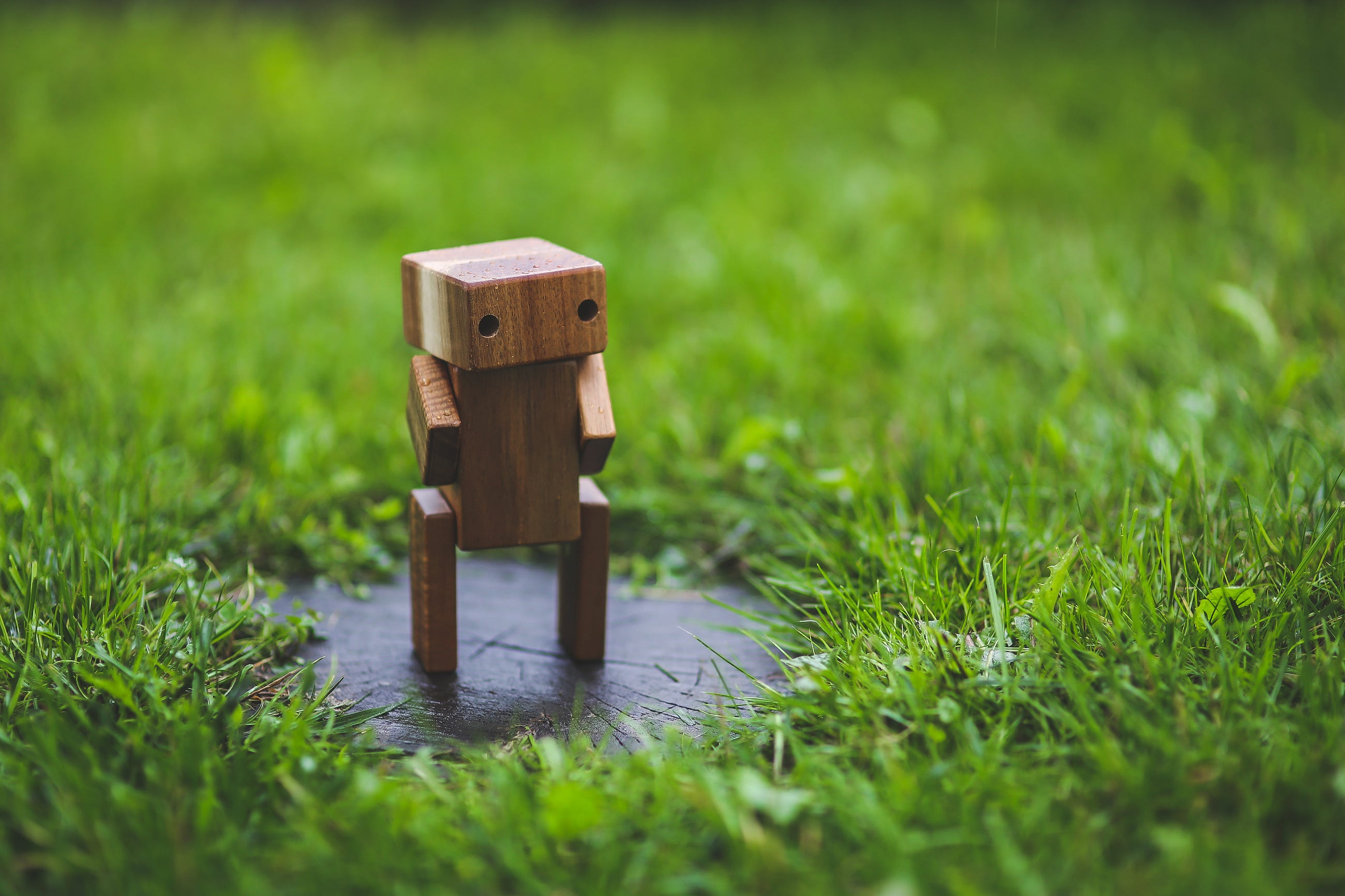# How Do Machines Learn?

Original article was published by Roman Paolucci on Artificial Intelligence on Medium# Artificial Intelligence

AI has turned into a buzzword everyone in the tech industry throws around, leaving the uninitiated mystified and in the dark. This article is meant to be an introduction to artificial intelligence and machine learning for those who may be unfamiliar with what it actually is, how it works, and what it can do.

Let’s get started…

# What is Data Science, AI, Machine Learning?

The realm of artificial intelligence is vast, however, there are certain subsections that narrow down its applications. Let’s start by introducing what is meant when we hear words like AI, ML, and Data Science. Whenever words like this are thrown around, generally, they are referring to processes where data that is either collected in the past or in realtime is being manipulated to create predictions. These predictions are the “end result” of the AI, ML, or some other statistical model after “seeing” the data. To better understand this let’s look at the three types of ways machines can learn…

• Supervised Learning
• Unsupervised Learning
• Reinforcement Learning

## Supervised Learning

When we have a large set (simply many observations of certain attributes) of data, let’s say height, weight, sex, and shoe size, we can use supervised learning to make predictions about certain attributes given others. Consider the example above, if we had a dataset of the following attributes…

…and we want to predict shoe size, we can use the height, weight, and sex of each individual in the dataset to help predict the shoe size (we will discuss how this process works below).

## Unsupervised Learning

Whenever we have a dataset where we are trying to identify unknown relationships we use unsupervised learning. That sounds almost like a contradicting statement doesn’t it?

Learn unknown relationships

Consider a data observed at a store with the following attributes…

• Sex
• Age
• Money Spent
• Time of Day

…what if we wanted to better understand who the top spenders were? More importantly what if there were key characteristics about top spenders that we could learn and then specifically target with marketing to get them in the door? That’s exactly what unsupervised learning aims to accomplish.

## Reinforcement Learning

Whenever we have an environment where rules can be learned, we can implement reinforcement learning. This is where the real fun starts with artificial intelligence — self-driving cars, AI’s capable of gaming, etc… In a sense, this is taken directly from psychology (in fact, most AI algorithms were inspired by human biology) where Pavlov trained a dog to salivate when hearing a bell, so too are AI’s trained to successfully complete their task based on a state-action-reward system.

# How Does a Machine Learn?

Let’s start by using linear regression to explain the basic steps in a linear model. First, let’s consider a dataset with the following arbitrary attributes…

`X1 X2 X3 X4 X5 X6 X7 X8 Y1 Y2`

In any instance, the X’s are given, and the Y’s are the outcome of the eight X variables (you can think of this as the shoe size example earlier). If we wanted to predict the Y’s based on the X’s the first step would be to separate the data into dependent (Y’s) and independent variables (X’s). Using python this would look like…

The data is now effectively split into two different sets…

• X — set of X1, X2, X3, … , X8
• Y — set of Y1 and Y2

Next, we can use a linear regression model to help predict set Y based on X…

So what happened here? Well, the linear regression model is just a line of best fit through the data. This means it’s the line where the distance from all the points to the line is minimized (in 2d). Which would look something like this…

This is how machines “learn”, they simply minimize the error of their predictions with observed outcomes

Now, what’s the issue with the linear regression model? Well, what the main assumption of a linear regression model is that there is a linear relationship between the dependent and independent variables. What if the data we plot looks more like this…

…obviously, there isn’t a linear relationship, it’s a non-linear relationship. This is where polynomial regression, neural networks, and all the other fun stuff comes into play. Unlike linear regression, a neural network can find non-linear relationships in data. This is why it is essential to understand the type of data you are working with and it’s relationship with the variable(s) you are trying to predict.

# Conclusion

In this article, I discussed different types of learning processes and gave an illustration as to how machines learn using a linear regression model. If you wish to learn more about the functionality of neural networks and how to build your own check out the following links…